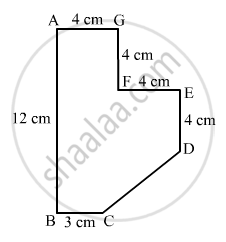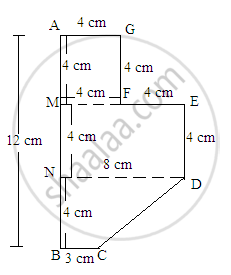Advertisement Remove all ads

# Find the Area of the Field Shown in Fig. 20.39 by Dividing It into a Square, a Rectangle and a Trapezium. - Mathematics

Sum

Find the area of the field shown in Fig. 20.39 by dividing it into a square, a rectangle and a trapezium.Advertisement Remove all ads

#### Solution

The given figure can be divided into a square, a parallelogram and a trapezium as shown in following figure:From the above figure:

Area of the figure=(Area of square AGFM with sides 4 cm)+(Area of rectangle MEDN with length 8 cm and width 4 cm)+(Area of trapezium NDCB with parallel sides 8 cm and 3 cm and perpendicular height 4 cm

$= (4\times4)+(8\times4)+[\frac{1}{2}\times(8+3)\times(4)]$

$= 16+32+22$

${= 70 cm}^2$

Is there an error in this question or solution?
Advertisement Remove all ads

#### APPEARS IN

RD Sharma Class 8 Maths
Chapter 20 Mensuration - I (Area of a Trapezium and a Polygon)
Exercise 20.2 | Q 20 | Page 24
Advertisement Remove all ads

#### Video TutorialsVIEW ALL 

Advertisement Remove all ads
Share
Notifications

View all notifications

Forgot password?
Course